• 在上一次多类别分类器练习中，通过多类别逻辑回归算法实现了识别数字笔迹。 但是，逻辑回归算法不能得到一个更为复杂的假设函数，因为它仅仅只是一个线性分类。 一，神经网络模型建立 建立模型示意图： 上图所...


神经网络
一，基于神经网络的多类别分类器1.1模型建立1.2前馈传播及多类别分类器的实现1.3代价函数
二、神经网络反向传播2.1sigmoid函数梯度2.2随机初始化2.3反向传播算法2.4梯度检测
三、优化参数

在上一次多类别分类器练习中，通过多类别逻辑回归算法实现了识别数字笔迹。 但是，逻辑回归算法不能得到一个更为复杂的假设函数，因为它仅仅只是一个线性分类，而神经网络能很好地实现这一点。 神经网路中最基本的成分是“神经元”模型，在生物神经网络中，每个神经元与其他神经元相连，当他“兴奋”时，就会向相连的神经元发送化学物质，从而改变这些神经元类的电位；如果某神经元的电位超过一个“阈值”，那么他就会被激活。神经元接收来自n个神经元传递过来的输入信号，这些输入信号通过带权重的连接进行传输，将神经元接收到的总输入值与神经元的自身的阈值进行比较，然后通过“激活函数”处理产生神经元的输出。理想中的激活函数是阶跃函数，但是它不连续，通常用sigmoid函数作为激活函数。
一，基于神经网络的多类别分类器
1.1模型建立
建立模型示意图：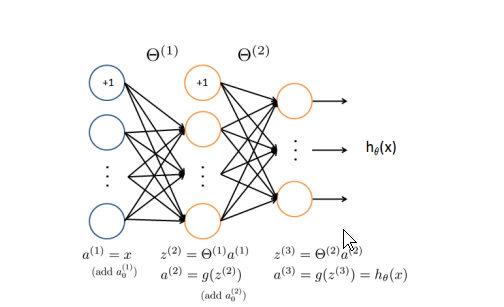上图所示神经网络一共有三个层，分别是输入层（input layer），隐藏层（hidden layer），输出层（output layer）。 特点如下： 1、每层由单元（units）组成 2、输入层是有训练集的实例特征向量传入 3、经过连接接点的权重（weight）传入下一层，一层的输出是下一层的输入 4、隐藏层的个数可以是任意的，输入层有一层，输出层有一层 5、每个单元也可以称之为神经结点，根据生物学来源定义 6、以上成为两层的神经网络，输入层是不算在里面的 7、一层中加权求和，然后根据非线性方程转化输出 8、作为多层向前神经网络，理论上，如果有足够的隐藏层，和足够的训练集，可以模拟出任何方程
1.2前馈传播及多类别分类器的实现
练习的数据集给出了实现数字字迹识别算法中需要的

θ

\theta

值，这里只需要运用神经网络前馈算法，利用已知的

θ

\theta

便可以轻松完成多类别分类器的实现。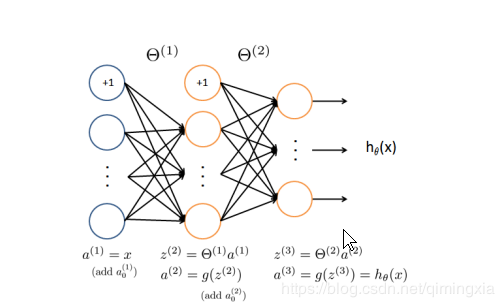上图的计算过程简写:

a

i

(

j

)

=

a_i^{(j)}=

第J层第i单元的“激活项”

θ

(

j

)

=

\theta^{(j)}=

控制函数从第J层映射到第J+1层的权重矩阵

a

1

(

2

)

=

g

(

θ

10

(

1

)

x

0

+

θ

11

(

1

)

x

1

+

θ

12

(

1

)

x

2

+

θ

13

(

1

)

x

3

)

a_1^{(2)}=g({\theta_{10}}^{(1)}x_0+{\theta_{11}}^{(1)}x_1+{\theta_{12}}^{(1)}x_2+{\theta_{13}}^{(1)}x_3)

a

2

(

2

)

=

g

(

θ

20

(

1

)

x

0

+

θ

21

(

1

)

x

1

+

θ

22

(

1

)

x

2

+

θ

23

(

1

)

x

3

)

a_2^{(2)}=g({\theta_{20}}^{(1)}x_0+{\theta_{21}}^{(1)}x_1+{\theta_{22}}^{(1)}x_2+{\theta_{23}}^{(1)}x_3)

a

3

(

2

)

=

g

(

θ

30

(

1

)

x

0

+

θ

31

(

1

)

x

1

+

θ

32

(

1

)

x

2

+

θ

33

(

1

)

x

3

)

a_3^{(2)}=g({\theta_{30}}^{(1)}x_0+{\theta_{31}}^{(1)}x_1+{\theta_{32}}^{(1)}x_2+{\theta_{33}}^{(1)}x_3)

…

h

θ

(

x

)

=

a

(

3

)

=

g

(

θ

(

2

)

x

0

+

θ

(

2

)

x

1

+

θ

(

2

)

x

2

+

θ

(

2

)

x

3

)

h_\theta(x)=a^{(3)}=g({\theta}^{(2)}x_0+{\theta}^{(2)}x_1+{\theta}^{(2)}x_2+{\theta}^{(2)}x_3)

… 令

z

1

(

2

)

=

θ

10

(

1

)

x

0

+

θ

11

(

1

)

x

1

+

θ

12

(

1

)

x

2

+

θ

13

(

1

)

x

3

z_1^{(2)}={\theta_{10}}^{(1)}x_0+{\theta_{11}}^{(1)}x_1+{\theta_{12}}^{(1)}x_2+{\theta_{13}}^{(1)}x_3

z

2

(

2

)

=

θ

20

(

1

)

x

0

+

θ

21

(

1

)

x

1

+

θ

22

(

1

)

x

2

+

θ

23

(

1

)

x

3

z_2^{(2)}={\theta_{20}}^{(1)}x_0+{\theta_{21}}^{(1)}x_1+{\theta_{22}}^{(1)}x_2+{\theta_{23}}^{(1)}x_3

… 其中g(z)是sigmoid函数
前向传播中“激活项”相关的代码实现
 def feed_forward(theta, X,):
'''得到每层的输入和输出'''
t1, t2 = deserialize(theta)

a1 = X
z2 = a1 @ t1.T
a2 = np.insert(sigmoid(z2), 0, 1, axis=1)
z3 = a2 @ t2.T
a3 = sigmoid(z3)

return a1, z2, a2, z3, a3

通过

θ

\theta

矩阵，可以由输入通过神经网络前向传播得到输出，即多类别分类器的代码实现：
import numpy as np
import pandas as pd
import matplotlib.pyplot as plt
from scipy.optimize import minimize

return data['Theta1'], data['Theta2']

def sigmoid(z):
return 1 / (1 + np.exp(-z))

X = data['X']
y = data['y']
return X,y

theta1.shape, theta2.shape

y = y.flatten()
X = np.insert(X, 0, values=np.ones(X.shape), axis=1)  # intercept

X.shape, y.shape
a1 = X
z2 = a1 @ theta1.T
z2.shape
z2 = np.insert(z2, 0, 1, axis=1)
a2 = sigmoid(z2)
a2.shape
z3 = a2 @ theta2.T
z3.shape
a3 = sigmoid(z3)
a3.shape
y_pred = np.argmax(a3, axis=1) + 1
accuracy = np.mean(y_pred == y)
print ('accuracy = {0}%'.format(accuracy * 100))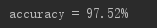可以看出基于神经网络的多类别分类器的准确率为0.9752。与上一篇博客中运用逻辑回归算法的效果要好。（逻辑回归算法得到的准确率为0.9446。）
1.3代价函数
已知逻辑回归的代价函数为：

J

(

θ

)

=

−

1

m

[

∑

i

=

1

m

y

(

i

)

log

⁡

(

h

θ

(

x

(

i

)

)

−

(

1

−

y

(

i

)

)

log

⁡

(

1

−

h

θ

(

x

(

i

)

)

)

]

+

λ

2

m

∑

j

=

1

n

θ

j

2

J(\theta)=-\frac{1}{m}[\sum_{i=1}^{m}y^{(i)}\log(h_\theta(x^{(i)})-(1-y^{(i)})\log(1-h_\theta(x^{(i)}))]+\frac{\lambda}{2m}\sum_{j=1}^{n}\theta_j^2

而神经网络的代价函数也是这个式子的一般形式，但这里不再仅有一个逻辑回归输出单元，取而代之的是存在K个。 所以，有神经网络的代价函数为:

J

(

θ

)

=

1

m

∑

i

=

1

m

∑

k

=

1

K

[

−

y

k

(

i

)

log

⁡

(

h

θ

(

x

k

(

i

)

)

−

(

1

−

y

k

(

i

)

)

(

log

⁡

(

1

−

h

θ

(

x

(

i

)

)

)

k

)

]

+

λ

2

m

∑

l

=

1

L

−

1

∑

i

=

1

S

l

∑

j

=

1

S

(

l

+

1

)

(

θ

(

j

i

)

(

l

)

)

2

J(\theta)=\frac{1}{m}\sum_{i=1}^{m}\sum_{k=1}^{K}[-y_k^{(i)}\log(h_\theta(x_k^{(i)})-(1-y_k^{(i)})(\log(1-h_\theta(x^{(i)}))_k)]+\frac{\lambda}{2m}\sum_{l=1}^{L-1}\sum_{i=1}^{S_l}\sum_{j=1}^{S_{(l+1)}}(\theta_{(ji)}^{(l)})^2

其中上图表示了

h

θ

(

x

(

i

)

)

h_\theta(x^{(i)})

的计算过程，K=10是可能的标签总数。注意，

h

θ

(

x

(

i

)

)

=

a

k

(

3

)

h_\theta(x^{(i)})=a_k^{(3)}

是第k个输出单元的激活(输出值)。此外，r 既然原来的标签(在变量y中)是1，2，…，10，为了训练神经网络，我们需要将标签重新编码为只包含值0或1的向量，即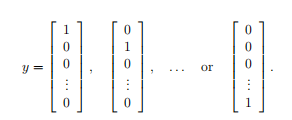例如，如果

x

(

i

)

x^{(i)}

是数字5的图像，最终得到的输出结果

y

(

i

)

y^{(i)}

应该是一个十维向量，其中元素

y

5

=

1

y_5=1

,并且其它元素的值为0。
def cost(theta, X, y):
a1, z2, a2, z3, h = feed_forward(theta, X)
J = 0
for i in range(len(X)):
first = - y[i] * np.log(h[i])
second = (1 - y[i]) * np.log(1 - h[i])
J = J + np.sum(first - second)
J = J / len(X)
return J
'''
# or just use verctorization
J = - y * np.log(h) - (1 - y) * np.log(1 - h)
return J.sum() / len(X)
'''
def regularized_cost(theta, X, y, l=1):
'''正则化时忽略每层的偏置项，也就是参数矩阵的第一列'''
t1, t2 = deserialize(theta)
reg = np.sum(t1[:,1:] ** 2) + np.sum(t2[:,1:] ** 2)  # or use np.power(a, 2)
return l / (2 * len(X)) * reg + cost(theta, X, y)


二、神经网络反向传播
在多类别分类器中，权重

θ

\theta

矩阵是给定的，能够轻松构建分类器。在线性回归，逻辑回归中可以用梯度下降法，或者其他优化算法得到代价函数收敛后的

θ

\theta

值。而在神经网络中，得到前馈传播权重的方法是反向传播。
2.1sigmoid函数梯度
sigmoid函数：

s

i

g

m

o

i

d

(

z

)

=

g

(

z

)

=

1

1

+

e

−

z

sigmoid(z)=g(z)=\frac{1}{1+{e}^{-z}}

它的导数

g

′

(

z

)

=

d

d

z

g

(

z

)

=

g

(

z

)

(

1

−

g

(

z

)

)

{g}&#x27;(z)=\frac{d}{dz}g(z)=g(z)(1-g(z))

公式用代码实现
def sigmoid_gradient(z):
return sigmoid(z) * (1 - sigmoid(z))

2.2随机初始化
当训练神经网络时，初始化参数的设置很重要。当设置的权重相同时，每层传播后得到的各个单元的数值相同，最后得到的y没有任何意义。所以需要随机初始化参数，破坏对称。 随机初始化的一种有效策略是在

[

−

ε

,

ε

]

[-\varepsilon,\varepsilon]

范围内均匀地为

Θ

(

l

)

\Theta^{(l)}

选择值。其中应该使用

ε

=

0.12

\varepsilon=0.12

。这个范围使得参数足够小，训练更有效率
def random_init(size):
'''从服从的均匀分布的范围中随机返回size大小的值'''
return np.random.uniform(-0.12, 0.12, size)

2.3反向传播算法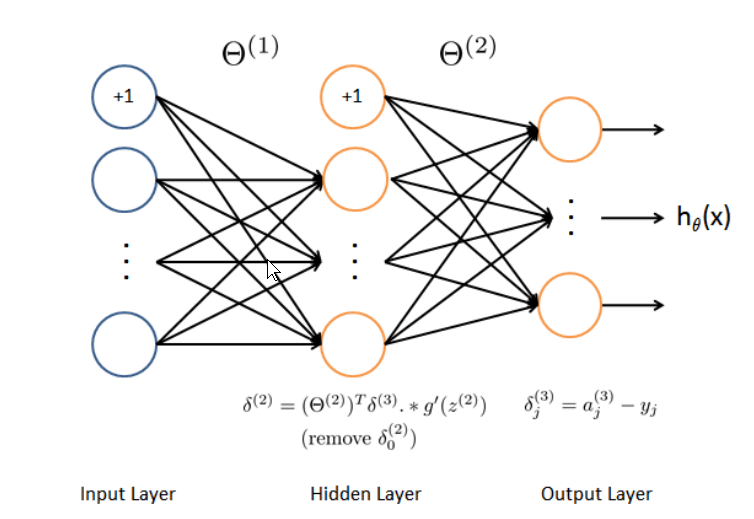根据已知训练集

(

x

(

t

)

,

y

(

t

)

)

(x^{(t)},y^{(t)})

,我们将利用神经网络前向传播，计算出整个网络中的“激活项”，以及最后的

h

Θ

(

x

)

h_\Theta(x)

.然后对于第

l

l

层的第

j

j

个节点，将计算出一个“误差项”

δ

j

(

l

)

\delta_j^{(l)}

,这衡量了该节点对我们输出中的任何错误“负责”的程度。 对于输出节点，我们可以直接测量网络的激活与真实目标值之间的差异，并且使用该差值来定义一个J(3)(因为层3是输出层)。对于隐藏层的 N个单位，将根据层(L+1)中节点的误差项的加权平均值来计算J(L)。 具体地，这里有反向传播算法(也在上图中示出)。 步骤: 1，将输入层的值(a(1))设置为第t个训练例x(t)。进行前向传播，计算第二层，第三层的“激活项”

(

z

(

2

)

,

a

(

2

)

,

z

(

3

)

,

a

(

3

)

)

(z^{(2)},a^{(2)},z^{(3)},a^{(3)})

。需要注意的是，需要添加一个1项，以确保层a(1)和a(2)的激活向量也包括偏置单元。
步骤： 2，对每一个输出层（第三层）的输出单元，设置

δ

k

(

3

)

=

(

a

k

(

3

)

−

y

k

)

\delta_k^{(3)}=(a_k^{(3)}-y_k)

, 其中，

y

k

∈

0

,

1

y_k\in{0,1}

,表示当前训练样本是否属于第K类（

y

k

=

1

y_k=1

），或者属于其他类（

y

k

=

0

y_k=0

）。
步骤： 3，对于隐藏层

（

l

=

2

）

（l=2）

,设置

δ

(

2

)

=

(

Θ

(

2

)

)

T

δ

(

3

)

.

∗

g

′

(

z

(

2

)

)

\delta^{(2)}=(\Theta^{(2)})^T\delta^{(3)}.*g^{&#x27;}(z^{(2)})

步骤： 4，计算

Δ

\Delta

值 对于训练集{

(

x

(

1

)

,

y

(

1

)

)

,

.

.

.

,

(

x

(

m

)

,

y

(

m

)

)

(x^{(1)},y^{(1)}),...,(x^{(m)},y^{(m)})

} 初始化设置

Δ

i

j

=

0

\Delta_{ij}=0

(

f

o

r

a

l

l

l

,

i

,

j

)

(for\space all \space l,i,j)

Δ

i

j

:

=

Δ

i

j

+

a

j

(

l

)

δ

i

(

l

+

1

)

\Delta_{ij}:=\Delta_{ij}+a_j^{(l)}\delta_i^{(l+1)}

步骤： 5，计算代价函数的梯度

D

i

j

(

l

)

:

=

1

/

m

Δ

i

j

(

l

)

+

λ

Θ

i

j

(

l

)

i

f

j

≠

0

D_{ij}^{(l)}:=1/m\Delta_{ij}^{(l)}+\lambda\Theta_{ij^{(l)}}\space\space\space if\space\space j\neq0

D

i

j

(

l

)

:

=

1

/

m

Δ

i

j

(

l

)

i

f

j

=

0

D_{ij}^{(l)}:=1/m\Delta_{ij}^{(l)}\space\space\space if\space\space j=0

∂

∂

θ

i

j

(

l

)

J

(

θ

)

=

D

i

j

(

l

)

\frac{\partial }{\partial \theta_{ij}^{(l)}}J(\theta)=D_{ij}^{(l)}

循环步骤1到4，最终得到代价函数的偏导数。 代码实现
def gradient(theta, X, y):
'''
unregularized gradient, notice no d1 since the input layer has no error
return 所有参数theta的梯度，故梯度D(i)和参数theta(i)同shape，重要。
'''
t1, t2 = deserialize(theta)
a1, z2, a2, z3, h = feed_forward(theta, X)
d3 = h - y # (5000, 10)
d2 = d3 @ t2[:,1:] * sigmoid_gradient(z2)  # (5000, 25)
D2 = d3.T @ a2  # (10, 26)
D1 = d2.T @ a1 # (25, 401)
D = (1 / len(X)) * serialize(D1, D2)  # (10285,)

return D

正则化
def regularized_cost(theta, X, y, l=1):
'''正则化时忽略每层的偏置项，也就是参数矩阵的第一列'''
t1, t2 = deserialize(theta)
reg = np.sum(t1[:,1:] ** 2) + np.sum(t2[:,1:] ** 2)  # or use np.power(a, 2)
return l / (2 * len(X)) * reg + cost(theta, X, y)


其中，deserialize函数功能是提取参数 当我们使用高级优化方法来优化神经网络时，我们需要将多个参数矩阵展开，才能传入优化函数，然后再恢复形状。
def serialize(a, b):
'''展开参数'''
return np.r_[a.flatten(),b.flatten()]

def deserialize(seq):
'''提取参数'''
return seq[:25*401].reshape(25, 401), seq[25*401:].reshape(10, 26)

2.4梯度检测
在神经网络算法中，希望得到最小的代价函数

J

(

θ

)

J(\theta)

。要对参数执行梯度检查，可以想象将参数Θ(1)、Θ(2)“展开”为长向量θ。通过这样做，可以考虑代价函数为J(θ)，并使用以下梯度检查过程。 假设有一个函数fi(θ)，该函数旨在计算

∂

∂

θ

i

J

(

θ

)

\frac{\partial }{\partial \theta_{i}}J(\theta)

；希望检查fi是否输出正确的导数值。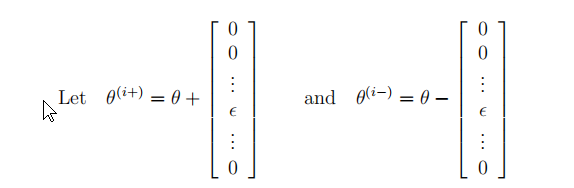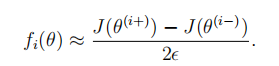这两个值的近似程度取决于J的细节，通过上式得到的结果去检测通过反向传播算法得到梯度。 代码实现
def gradient_checking(theta, X, y, e):
"""
对每个参数theta_i计算数值梯度，即理论梯度。
"""
return (regularized_cost(plus, X, y) - regularized_cost(minus, X, y)) / (e * 2)

for i in range(len(theta)):
plus = theta.copy()  # deep copy otherwise you will change the raw theta
minus = theta.copy()
plus[i] = plus[i] + e
minus[i] = minus[i] - e

print('If your backpropagation implementation is correct,\nthe relative difference will be smaller than 10e-9 (assume epsilon=0.0001).\nRelative Difference: {}\n'.format(diff))

三、优化参数
使用minimize函数优化参数 当隐藏层变多的时候我们的损失函数就变成了一个非凸函数如下图所示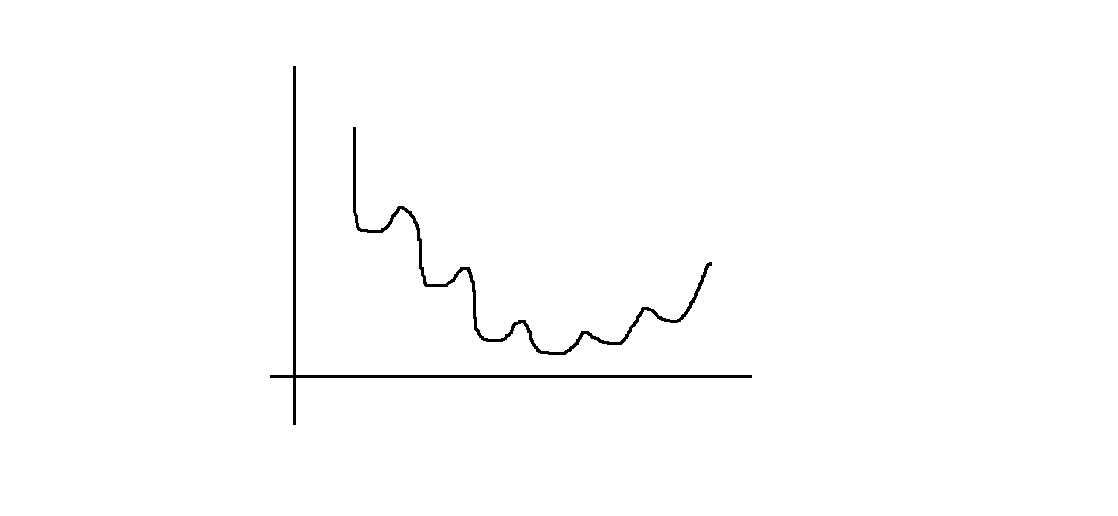这是考虑使用minimize进行非线性规划
scipy.optimize.minimize(fun, x0, args=(), method=None, jac=None, hess=None, hessp=None, bounds=None, constraints=(), tol=None, callback=None, options=None)

fun: 求最小值的目标函数
x0: 变量的初始猜测值，如果有多个变量，需要给每个变量一个初始猜测值。minimize是局部最优的解法，所以
args: 常数值,后面demo会讲解，fun中没有数字，都以变量的形式表示，对于常数项，需要在这里给值
method: 求极值的方法，官方文档给了很多种。一般使用默认。每种方法我理解是计算误差，反向传播的方式不同而已，这块有很大理论研究空间
constraints: 约束条件，针对fun中为参数的部分进行约束限制
def nn_training(X, y):
init_theta = random_init(10285)  # 25*401 + 10*26

res = opt.minimize(fun=regularized_cost,
x0=init_theta,
args=(X, y, 1),
method='TNC',
options={'maxiter': 400})
return res

res = nn_training(X, y)#慢
res
'''
fun: 0.5156784004838036
jac: array([-2.51032294e-04, -2.11248326e-12,  4.38829369e-13, ...,
9.88299811e-05, -2.59923586e-03, -8.52351187e-04])
message: 'Converged (|f_n-f_(n-1)| ~= 0)'
nfev: 271
nit: 17
status: 1
success: True
x: array([ 0.58440213, -0.02013683,  0.1118854 , ..., -2.8959637 ,
1.85893941, -2.78756836])
'''

def accuracy(theta, X, y):
_, _, _, _, h = feed_forward(res.x, X)
y_pred = np.argmax(h, axis=1) + 1
print(classification_report(y, y_pred))

accuracy(res.x, X, raw_y)
'''
precision    recall  f1-score   support

1       0.97      0.99      0.98       500
2       0.98      0.97      0.98       500
3       0.98      0.95      0.96       500
4       0.98      0.97      0.97       500
5       0.97      0.98      0.97       500
6       0.99      0.98      0.98       500
7       0.99      0.97      0.98       500
8       0.96      0.98      0.97       500
9       0.97      0.98      0.97       500
10       0.99      0.99      0.99       500

avg / total       0.98      0.98      0.98      5000
'''


展开全文• 吴恩达老师机器学习课的笔记。黄海广博士原创，黄海广博士也是这门课的最初翻译者。包括课堂笔记，数学基础，Python代码
• 对于二元分类，上一篇博课已经给出分析：二元分类原理分析，及代码实现过程 运用逻辑回归，很好地实现了分类的功能。 对于一个多分类问题，以三元分类为例，建立数据模型如下， 使用三种符号，...


多类别分类
一、模型建立二、一对多分类方法(one-vs-all)三、分类器实现1，加载数据集（Dateset），可视化2，向量化逻辑回归2.1向量化正则化的代价函数2.2向量化梯度
3，一对多分类器（one-vs-all）4，One-vs-all Prediction

一、模型建立
对于二元分类的数据模型如下；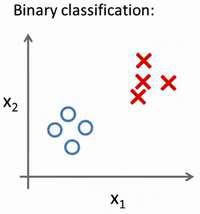使用两种符号表示两个不同的数据集。 对于二元分类，上一篇博客已经给出分析：二元分类原理分析，及代码实现过程 运用逻辑回归，很好地实现了分类的功能。 对于一个多分类问题，以三元分类为例，建立数据模型如下，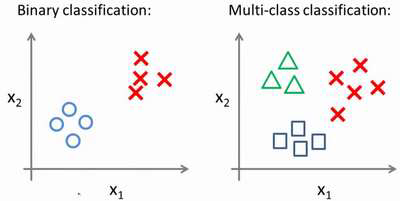使用三种符号，表示三种不同类别的数据集。
现在已经知道如何进行二元分类，可以使用逻辑回归，对于直线，同时可以将数据集一分为二为正类和负类。用一对多的分类思想，我们可以将其用在多类分类问题上。有时这个方法也被称为"一对余"(one-vs-rest)方法。
二、一对多分类方法(one-vs-all)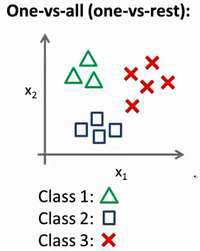以三元分类为例，有一个训练集，好比上图表示的有3 个类别，我们用三角形表示 ? = 1，方框表示? = 2，叉叉表示 ? = 3。通过使用一个训练集，将其分成3 个二元分类问题。 　　先从用三角形代表的类别1 开始，实际上可以创建一个，新的"伪"训练集，类型2 和类型3 定为负类，类型1 设定为正类，创建一个新的训练集，如下图所示的那样，要拟合出一个合适的分类器。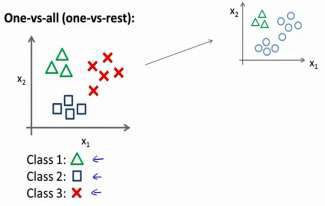这里的三角形是正样本，而圆形代表负样本。可以这样想，设置三角形的值为1，圆形的值为0，下面来训练一个标准的逻辑回归分类器，这样我们就得到一个正边界。为了能实现这样的转变，我们将多个类中的一个类标记为正向类（? = 1），然后将其他所有类都标记为负向类，这个模型记作

h

θ

1

(

x

)

h_{\theta1}(x)

;接着，类似地选择另一个类标记为正向类（? = 2），再将其它类都标记为负向类，将这个模型记作

h

θ

2

(

x

)

h_{\theta2}(x)

;依此类推。 　　最后得到一系列的模型简记为：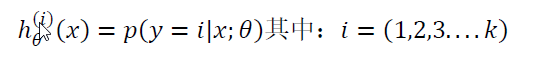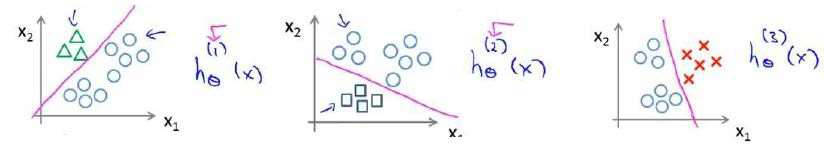最后，在需要做预测时，将所有的分类机都运行一遍，然后对每一个输入变量，都选择最高可能性的输出变量。
三、分类器实现
以数字字迹识别为例，讨论多类别分类问题。
1，加载数据集（Dateset），可视化
def load_data(path):
X = data['X']
y = data['y']
return X,y


X, y = load_data('ex3data1.mat')
print(np.unique(y))  # 看下有几类标签
# [ 1  2  3  4  5  6  7  8  9 10]
X.shape, y.shape
# ((5000, 400), (5000, 1))


控制台输出数据中的类别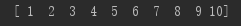其中有5000个训练样本，每个样本是20*20像素的数字的灰度图像。每个像素代表一个浮点数，表示该位置的灰度强度。20×20的像素网格被展开成一个400维的向量。在我们的数据矩阵X中，每一个样本都变成了一行，这给了我们一个5000×400矩阵X，每一行都是一个手写数字图像的训练样本。 由Y数组，查看得到数据集分为，[1,2,3,4,5,6,7,8,9,10]十个类别。 随机查看1个训练样本，查看100个训练样本。
def plot_an_image(X):
"""
随机打印一个数字
"""
pick_one = np.random.randint(0, 5000)
image = X[pick_one, :]
fig, ax = plt.subplots(figsize=(1, 1))
ax.matshow(image.reshape((20, 20)), cmap='gray_r')
plt.xticks([])  # 去除刻度，美观
plt.yticks([])
plt.show()
print('this should be {}'.format(y[pick_one]))

def plot_100_image(X):
"""
随机画100个数字
"""
sample_idx = np.random.choice(np.arange(X.shape), 100)  # 随机选100个样本
sample_images = X[sample_idx, :]  # (100,400)

fig, ax_array = plt.subplots(nrows=10, ncols=10, sharey=True, sharex=True, figsize=(8, 8))

for row in range(10):
for column in range(10):
ax_array[row, column].matshow(sample_images[10 * row + column].reshape((20, 20)),
cmap='gray_r')
plt.xticks([])
plt.yticks([])
plt.show()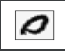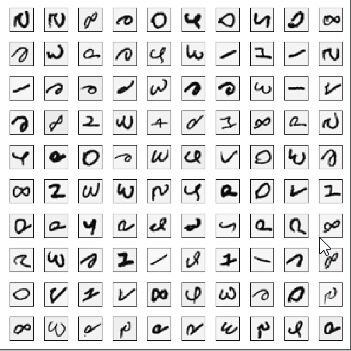2，向量化逻辑回归
使用多个one-vs-all(一对多)logistic回归模型来构建一个多类别分类器。由于有10个类，需要训练10个独立的分类器。为了提高训练效率，重要的是向量化。
2.1向量化正则化的代价函数
正则化的logistic回归的代价函数是：

J

(

θ

)

=

−

1

m

[

∑

i

=

1

m

y

(

i

)

log

⁡

(

h

θ

(

x

(

i

)

)

−

(

1

−

y

(

i

)

)

log

⁡

(

1

−

h

θ

(

x

(

i

)

)

)

]

+

λ

2

m

∑

j

=

1

n

θ

j

2

J(\theta)=-\frac{1}{m}[\sum_{i=1}^{m}y^{(i)}\log(h_\theta(x^{(i)})-(1-y^{(i)})\log(1-h_\theta(x^{(i)}))]+\frac{\lambda}{2m}\sum_{j=1}^{n}\theta_j^2

对于每个样本

i

i

要计算，

h

θ

(

x

(

i

)

)

h_\theta(x^{(i)})

,

h

θ

(

x

(

i

)

)

=

g

(

θ

T

x

(

i

)

)

h_\theta(x^{(i)})=g(\theta^Tx^{(i)})

,

g

(

z

)

g(z)

是sigmoid函数 事实上我们可以对所有的样本用矩阵乘法来快速的计算。 定义X，与

θ

\theta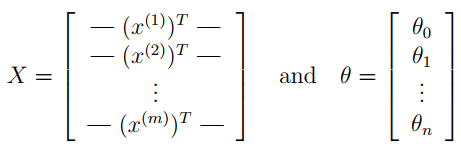通过计算X

θ

\theta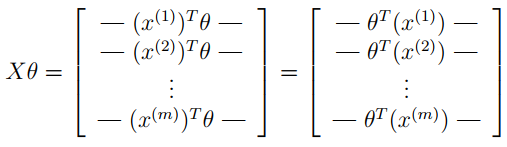在最后一个等式中，我们用到了一个定理，如果

a

a

和

b

b

都是向量，那么

a

T

b

=

b

T

a

a^Tb=b^Ta

这样我们就可以用一行代码计算出所有的样本。
def sigmoid(z):
return 1 / (1 + np.exp(-z))

def regularized_cost(theta, X, y, l):
"""
don't penalize theta_0
args:
X: feature matrix, (m, n+1) # 插入了x0=1
y: target vector, (m, )
l: lambda constant for regularization
"""
thetaReg = theta[1:]
first = (-y*np.log(sigmoid(X@theta))) + (y-1)*np.log(1-sigmoid(X@theta))
reg = (thetaReg@thetaReg)*l / (2*len(X))
return np.mean(first) + reg


2.2向量化梯度
未正则化逻辑回归代价函数的梯度为：

∂

∂

/

θ

j

J

(

θ

)

=

−

1

m

∑

i

=

1

m

(

h

θ

(

x

(

i

)

)

−

y

(

i

)

)

x

j

(

i

)

\frac{\partial }{\partial /\theta_j}J(\theta)=-\frac{1}{m}\sum_{i=1}^{m} (h_\theta(x^{(i)})-y^{(i)})x_j^{(i)}

使用向量化的方法表示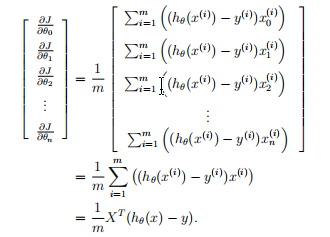其中，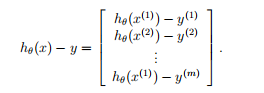注意，

h

θ

(

x

(

i

)

)

−

y

(

i

)

h_\theta(x^{(i)})-y^{(i)}

是一个标量，令

β

i

=

h

θ

(

x

(

i

)

)

−

y

(

i

)

\beta_i=h_\theta(x^{(i)})-y^{(i)}

得到：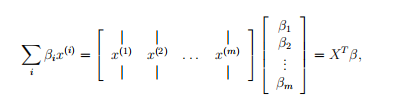正则化后的梯度下降算法表示如下，不惩罚

θ

0

\theta_0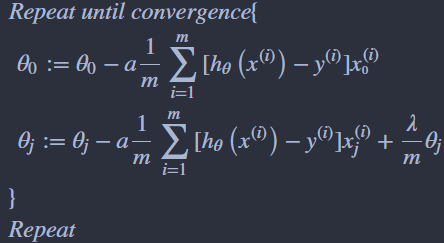代码实现
def regularized_gradient(theta, X, y, l):
"""
don't penalize theta_0
args:
l: lambda constant
return:
"""
thetaReg = theta[1:]
first = (1 / len(X)) * X.T @ (sigmoid(X @ theta) - y)
# 这里人为插入一维0，使得对theta_0不惩罚，方便计算
reg = np.concatenate([np.array(), (l / len(X)) * thetaReg])
return first + reg

3，一对多分类器（one-vs-all）
这部分通过训练多个正则化logistic回归分类器实现一对多分类，每个分类器对应数据集中K类中的一个。 对于这个任务，我们有10个可能的类，并且由于logistic回归只能一次在2个类之间进行分类，每个分类器在“类别 i”和“不是 i”之间决定。 我们将把分类器训练包含在一个函数中，该函数计算10个分类器中的每个分类器的最终权重，并将权重返回shape为(k, (n+1))数组，其中 n 是参数数量。
from scipy.optimize import minimize

def one_vs_all(X, y, l, K):
"""generalized logistic regression
args:
X: feature matrix, (m, n+1) # with incercept x0=1
y: target vector, (m, )
l: lambda constant for regularization
K: numbel of labels
return: trained parameters
"""
all_theta = np.zeros((K, X.shape))  # (10, 401)

for i in range(1, K+1):
theta = np.zeros(X.shape)
y_i = np.array([1 if label == i else 0 for label in y])

ret = minimize(fun=regularized_cost, x0=theta, args=(X, y_i, l), method='TNC',
all_theta[i-1,:] = ret.x

return all_theta


这里需要注意的几点：首先，我们为X添加了一列常数项 1 ，以计算截距项（常数项）。 其次，我们将y从类标签转换为每个分类器的二进制值（要么是类i，要么不是类i）。 最后，我们使用SciPy的较新优化API来最小化每个分类器的代价函数。 如果指定的话，API将采用目标函数，初始参数集，优化方法和jacobian（渐变）函数。 然后将优化程序找到的参数分配给参数数组。
4，One-vs-all Prediction
在训练多类别分类器之后，现在可以用它来预测给定图像中包含的数字。对于每个输入，您应该使用以下方法计算它属于每个类的“概率” 训练的Logistic回归分类器。One-vs-all预测函数将选择相应的逻辑回归分类器输出概率最高的类和ret。 将类标签(1、2、…或K)作为输入示例的预测。 实现向量化代码的一个更具挑战性的部分是正确地写入所有的矩阵，保证维度正确。
def predict_all(X, all_theta):
# compute the class probability for each class on each training instance
h = sigmoid(X @ all_theta.T)  # 注意的这里的all_theta需要转置
# create array of the index with the maximum probability
# Returns the indices of the maximum values along an axis.
h_argmax = np.argmax(h, axis=1)
# because our array was zero-indexed we need to add one for the true label prediction
h_argmax = h_argmax + 1

return h_argmax


这里的h共5000行，10列，每行代表一个样本，每列是预测对应数字的概率。我们取概率最大对应的index加1就是我们分类器最终预测出来的类别。返回的h_argmax是一个array，包含5000个样本对应的预测值。
raw_X, raw_y = load_data('ex3data1.mat')
X = np.insert(raw_X, 0, 1, axis=1) # (5000, 401)
y = raw_y.flatten()  # 这里消除了一个维度，方便后面的计算 or .reshape(-1) （5000，）

all_theta = one_vs_all(X, y, 1, 10)
all_theta  # 每一行是一个分类器的一组参数
y_pred = predict_all(X, all_theta)
accuracy = np.mean(y_pred == y)
print ('accuracy = {0}%'.format(accuracy * 100))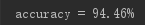通过预测的y值与实际y值进行比对，得出结果在这次练习中的多类别分类器的准确率是0.9446。
展开全文机器学习
• 本文件是网易云课堂吴恩达老师机器学习课程课后作业的Python实现，本部分是线性回归的作业，其他部分的作用也会陆续上传。
• 新智元报道 来源：Reddit、GitHub编辑：肖琴【导读】完全用Python完成吴恩达的机器学习课程是怎样一种体验？本文作者表示：完全可以！而且你不需要成为Pyt...新智元报道
来源：Reddit、GitHub
编辑：肖琴
【导读】完全用Python完成吴恩达的机器学习课程是怎样一种体验？本文作者表示：完全可以！而且你不需要成为Python专家，只需要有良好的数学基础。

“吴恩达的Coursera机器学习课程可以完全用Python完成！”

昨天，Reddit论坛的机器学习看板出现这样一条热帖。

可以说，吴恩达（Andrew Ng）的机器学习课程是很多人、尤其是中国学生进入机器学习世界的引路人，被认为是入门机器学习的最好课程。截至目前，有超过80000人在Coursera上完成了这门课的学习。吴恩达Machine Learning课的评分

不过，这门课推荐使用Matlab/Octave来完成作业，对于不会Matlab/Octave，或者对Matlab/Octave不感兴趣的人来说，要完成作业获取证书可能难度加大。虽然吴恩达强烈推荐大家使用 Octave 来学习机器学习，并且用了完整的一个章节“Octave and Matlab Tutorial”来讲述 Octave 的基本操作。

那么，全部用Python完成是怎么回事？

Reddit用户rsdsdsr的帖子如下：

一句话总结：吴恩达的Coursera ML课程可以用Python完成，而且你不必是Python专家就可以做到。但你需要有很好的数学基础，这将是非常耗时的，而且用Python写作业不会得到证书。”

我最近在Coursera上完成了吴恩达的ML课程，但我没有使用推荐的Matlab/Octave，而是完全用Python来完成。你可以在这里查看我作业的Jupyter notebooks：
https://github.com/seddonr/Ng_ML

我绝不是第一个这样做的人，但我认为应该发这个帖子，作为给那些正在考虑参加这门课程，但像我一样对学习Matlab/Octave不感兴趣的人的鼓励，也可以了解用Python学这门课的好处和缺点。

用Python完成这门课程非常耗时，因为这意味着我必须从头开始构建所有东西，而如果你用Matlab/Octave完成课程，则会得到大量预编写的代码。另一方面，这是一次很棒的学习经历，我学到了大量的Python技能。

你不必成为Python专家——在完成这门课程之前，我的Python经验仅限于“使用Python自动化枯燥的东西”，以及学过一些关于NumPy和Matplotlib基础知识的非常简短的教程。但是，你需要有良好的数学基础才能跟上课程——有很多矩阵代数和微积分。

另一个问题是，由于这门课程是为Matlab/Octave设计的，因此无法提交用Python完成的作业，所以你无法对你的作业进行评分或获得证书。证书对我来说并不重要，但如果对你很重要，那么Matlab/Octave可能是更好的选择。

总的来说，我觉得这门课程很有趣，也很享受，我觉得它很好地覆盖了ML的许多主题。 Andrew Ng能以一种愉快的语调把知识点讲得很清楚，他的课也很容易学。如果你决定尝试用Python完成这门课程，祝你好运，我希望这个帖子是令人鼓舞的！

Python作业代码

rsdsdsr公开了他的作业代码：
https://github.com/seddonr/Ng_ML

内容：
Ex1：线性回归
有一个变量和多个变量。

Ex2：逻辑回归
包括正则化。

Ex3： Multi-class 分类和神经网络
使用两种不同的方法识别手写数字：one-vs-all逻辑回归，以及前向传播预训练的神经网络。

Ex4：神经网络学习
构建和训练神经网络，包括反向传播，并将其用于手写数字识别。

Ex5：正则化线性回归和偏差与方差
包括学习曲线和多项式回归。

Ex6：支持向量机
6.1：在一些样本2D数据集上展示scikit-learn SVM，其中包含线性和高斯核函数。
6.2：使用自然语言处理和scikit-learn SVM构建电子邮件垃圾邮件分类器。

Ex7：K-means聚类和主成分分析
7.1：构建k-means聚类算法并将其用于图像压缩。
7.2：构建PCA算法并将其用于图像压缩和可视化。

Ex8：异常检测和推荐系统
8.1：使用多元高斯模型进行异常检测。 Precision, recall, F1 score.8.2：构建推荐系统并用它来推荐电影。
最佳入门教程

吴恩达在Coursera上开设的Machine Learning课程，经过数年的改进和传播，目前已有许多中文学习资料。吴恩达本人对这门课也很有感情，他曾表示自己保留斯坦福教职，很大程度上是因为想教这门课。

这门课也是技巧和实践并重，“你不仅可以学习到学习的理论基础，还可以获得所需的实用知识，快速有效地将这些技术应用于新的问题”。课程中还将介绍了硅谷在机器学习和人工智能方面创新的一些最佳实践。本课程还将从大量的案例研究和应用中吸取教训，以便学习如何将学习算法应用于构建智能机器人（感知，控制），文本理解（网络搜索，反垃圾邮件），计算机视觉，医学信息学，音频，数据库挖掘等领域。

主题包括：
1、监督学习：参数/非参数算法，支持向量机，Kernels，神经网络
2、无监督学习：聚类，降维，推荐系统，深度学习
3、机器学习的最佳实践：偏差/方差理论；机器学习和人工智能的创新过程

最后，该课最新一期从10月15日开始，持续约11周，2019年1月7日结束。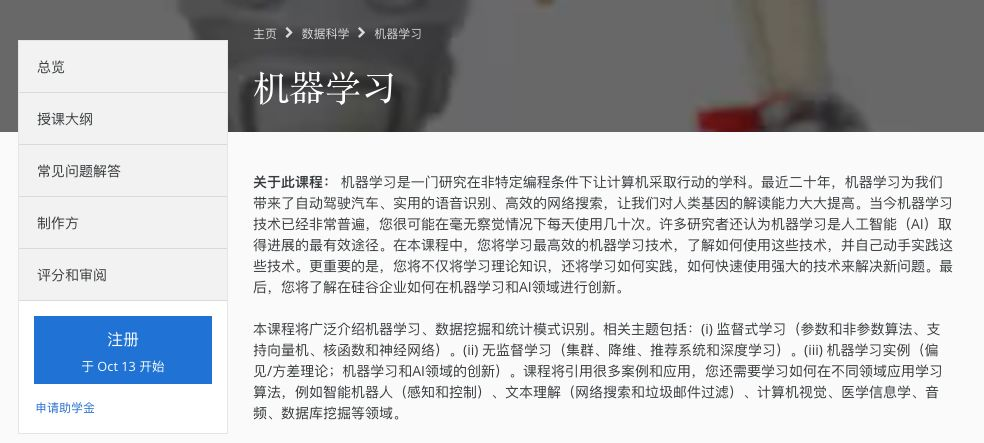课程地址：
https://www.coursera.org/learn/machine-learning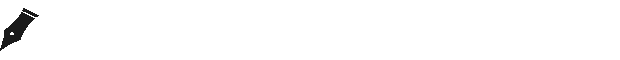点击下方“阅读原文”了解【人工智能服务器】
↓↓↓


展开全文• 吴恩达线性回归作业python实现，希望对后面学习的同学有所帮助
• 为了分享和帮助广大同学在学习吴恩达机器学习课程的同时，在缺少linux环境下，用pycharm编程实现代码，本资源提供了机器学习代码python版，并有详细注释，原有来源“黄海广”
• 吴恩达机器学习作业的Python实现，该部分是神经网络的作业，里面包含数据集和代码
• 吴恩达深度学习作业代码，包括全部作业代码Python实现，是官方的代码答案，包括所有的作业代码和讲解。
• 吴恩达机器学习作业Python版 主要记录自己学习机器学习的过程，以及自己看别人代码时不懂或者疑惑的地方，代码主要参考Cowry5作者的代码，原作者代码和讲解更为详细，本文章如有不好的地方，还请去浏览大神的原文章...
吴恩达机器学习作业Python版
主要记录自己学习机器学习的过程，以及自己看别人代码时不懂或者疑惑的地方，代码主要参考Cowry5作者的代码，原作者代码和讲解更为详细，本文章如有不好的地方，还请去浏览大神的原文章(https://blog.csdn.net/Cowry5)
线性回归（一）
单变量线性回归
题目要求：自己实现单变量线性回归，以预测食品卡车的利润
导入数据：
import numpy as np
import pandas as pd

#读取文件
print("data的维度为：")
print(data.shape,"\n") #检查维度，期待输出（97，2）

变量初始化： 根据吴恩达老师所讲，为便于计算，我们在矩阵最前列加入全“1”列，然后取矩阵前两列为x（输入矩阵），最后一列为y（输出矩阵）
data.insert(0,'ones',1)
x=data.iloc[:,:-1].values
y=data.iloc[:,-1].values #.values方法即为将数据转化为矩阵形式
theta=np.zeros(x.shape)
print("x,y,theta的维度分别为:")
print(x.shape,y.shape,theta.shape,"\n") #检查维度，期待输出（97，2），（97，），（2，）
#插入全1列便于后续矩阵计算， 取读取数据的前两列为x矩阵（即为输入矩阵),最后一列为y矩阵（输出矩阵）

定义代价函数
#定义代价函数
def calculateCost(x,y,theta):
cost=np.sum((x@theta-y)**2)/(2*len(x)) #@为矩阵乘法符号
return cost

定义梯度下降函数
#定义梯度下降函数
tmp=np.zeros(x.shape)
i=1
#Cowry5大神的方法为取1000次计算后的结果为最终结果
#但我觉得那样数据可能不够精确，所以我定义一个永真循环
#一直计算，直至theta不在发生变化，打破循环
while(True):
tmp = theta
theta = theta - a * (1 / len(x)) * x.T @ (x @ theta - y)
if((tmp==theta).all()):
print("calculation is done!")
print("计算次数为：",i,"次\n")
break
i+=1
return theta

调用方法，输出结果：

print("结果显示：")
print("最终theta为：",theta)
print("最终最小代价为：",calculateCost(x,y,theta))

最终结果输出相比于计算1000次得到的最终代价：4.515955503078914，永真循环计算得到的结果更为精确。
注：最后放上整个文件的连接，里面有完整代码和数据，谢谢大家！ （^ __ ^）
链接1：csdn资源
链接2：百度云网盘 提取码：hhnb 复制这段内容后打开百度网盘手机App，操作更方便哦
展开全文机器学习 人工智能
• Python版本3.6 编译环境：anaconda 需要数据集ex1data1.txt、ex1data2.txt 或者 编程作业ex1.pdf在评论区留下邮箱！ 1 单变量线性回归(Linear regression with one variable) 本章课程笔记部分见：单变量线性...机器学习 线性回归
• 在本部分的练习中，您将使用一个变量实现线性回归，以预测食品卡车的利润。假设你是一家餐馆的首席执行官，正在考虑不同的城市开设一个新的分店。该连锁店已经在各个城市拥有卡车，而且你有来自城市的利润和人口数据...
• 吴恩达老师的机器学习作业。包含作业描述，Python实现和数据集。作业描述是英文的，比较简单大家可以看懂
• 这是Coursera网站上，课程中算法在Matlab语言的实现，同样也可以参考斯坦福大学的计算机课程 。 注意： 您可以在上下载完整课程视频和相关文档； 定义 “如果某计算机程序在T上的性能（由P来衡量）随着经验E的提高而...
• 吴恩达机器学习编程作业python3.6版本，编译环境pycharm，配置Anaconda3 。代码含有较为详细的注释，对初学者较合适。
• 吴恩达深度学习课后作业/第一门课第四周作业2，亲测跑通版，含数据集、Python代码及程序正确跑通视频录像，实现正确识别分类和错误图像的输出，与网上的不太一样，这个属于自己微调的版本；
• 里面可以下载进行代码实现的数据集data.txt或data.csv 介绍： 最近在看吴恩达机器学习视频的时候，为了巩固自己的基础，想亲自实现一下课程里面的每个算法。在实现之前，我先看了一下别人实现的相关代码，看完就...机器学习 算法 线性回归
• ex I-代码实现 单变量线性回归 在本部分的练习中，您将使用一个变量实现线性回归，以预测食品卡车的利润。假设你是一家餐馆的首席执行官，正在考虑不同的城市开设一个新的分店。该连锁店已经在各个城市拥有卡车，...机器学习 人工智能
• Coursera_ML_Exercise 吴恩达机器学习公开课作业中文版本 几点说明 ...每个文件夹中.md文件为翻译的中文作业，.ipynb为作业的Python代码实现。Python代码是在黄海广博士以及的代码基础上做了部分修改。
• ## 吴恩达机器学习课程全(python实现)

千次阅读 多人点赞 2020-03-03 17:20:32
吴恩达机器学习课程(python实现) 作业1-线性回归 作业2-逻辑回归 作业3-多分类和神经网络 作业4-神经网络训练 作业5-Bias vs Variance 作业6-支持向量机 完成作业时参考了博主Cowry5，在这里表示感谢！ 文章中...机器学习
• 吴恩达机器学习资料获取 单变量线性回归 问题背景：假如你是餐馆老板，已知若干城市中人口和利润的数据（ex1data1.txt），用线性回归方法计算该去哪个城市发展。 #导入 import numpy as np import pandas as pd ...机器学习 数据挖掘
• ∗∗∗ 点击查看 :吴恩达机器...本次作业的理论部分：吴恩达机器学习（七）支持向量机 编程环境：Jupyter Notebook 1. 线性 SVM 任务 观察惩罚项系数 C 对决策边界的影响，数据集：data/ex6data1.mat 在理论部分，
• 主要根据吴恩达的机器学习视频来学习梯度下降算法，并用代码实现。 梯度下降算法的目的是求使代价函数最小的θ的值，附上相关公式。 上图是假设函数和代价函数的定义，而梯度下降算法的目的就是为了找到是代价...机器学习 梯度下降算法
• Population Size') plt.show() 源码： import numpy as np import pandas as pd import matplotlib.pyplot as plt path = 'D:\工作相关\参考资料\吴恩达\机器学习\Coursera-ML-AndrewNg-Notes-master\code\ex1-...
• 实现线性回归算法2.1 初始化参数2.2 实现损失函数2.3 实现梯度下降2.4 将得到的直线绘制出来,观察拟合程度3.完整代码 1.数据处理部分 1.1 加载数据 data_file_path = "ex1/ex1data1.txt" # 此处需根据你的数据文件的...机器学习 编程作业
• 内有详细搜集的吴恩达机器学习课的课后习题编程实现代码，有官方提供的matlab版本，还有多个用python实现的版本，并且附有详细的注释以及作业文档说明。
• 代码使用matlab来实现，且只需要填充一些关键步骤，很容易漏掉一些信息，故而用python实现一下：前半部分：# -*- coding...说明：本文档根据吴恩达机器学习课后作业改编而成，源代码是matlab ''' import numpy as ......

# 吴恩达python代码实现python 订阅